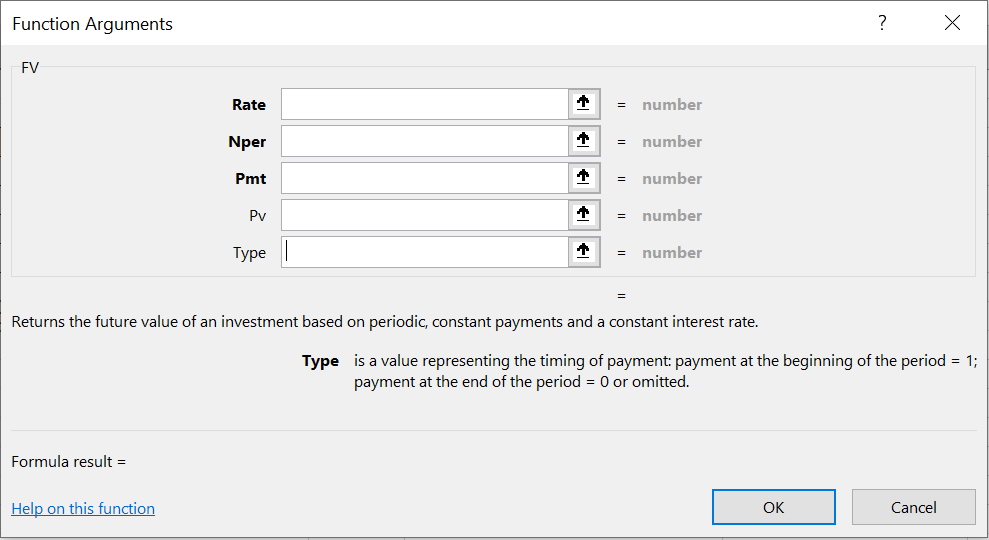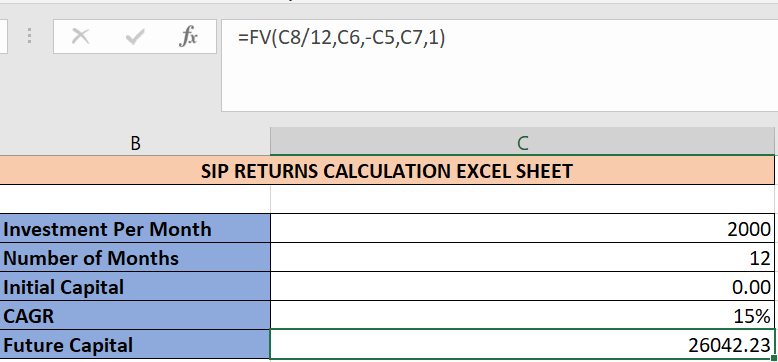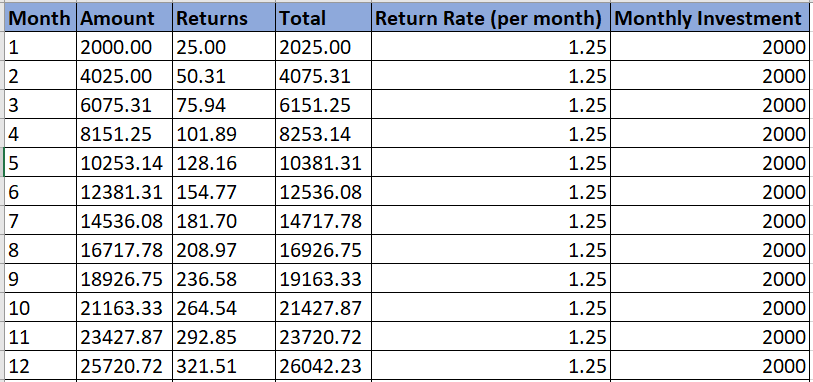# How to Calculate SIP Returns in an Excel Sheet?

Most of the modern-day investors prefer a systematic investment plan (SIP) to invest their funds at regular intervals. And it is a really smart way to average out your investment during different phases of the market. On a long-term SIP always yields good returns. You can do SIP in mutual funds as well as stocks, though it’s more popular among mutual fund investors. In this post, we’ll learn how to calculate SIP returns in an Excel sheet.

## Calculate SIP Returns through Excel FV Function

The final value function accepts 5 arguments, of which 3 are mandatory.1. Rate: It’s the rate of return for the period specified. For example: if you are expecting 15% per annum return for your mutual fund SIP, then the rate would be 15/12=1.25% per month
2. Nper: Number of periods for which investment is made. So, if you invest a certain amount every month for 5 years, then Nper would be 5*12 = 60
3. Pmt: Investment per period, can also be called a SIP amount. It is expressed in negative as it represents the net outflow of money.
4. Pv: Initial invested amount before the SIP is started. Default is 0
5. Type: Specifies when the investment is made for a particular period. 1 denote the beginning of the period, 0 denotes the end of the period.

See below how this function is put to use in the excel sheet to calculate the final capital for a SIP investment with 15% per annum return.This function is very useful and abstracts a lot of complexity from the users.

## Calculate SIP Returns manually

The only drawback of using the FV function to calculate SIP returns is that you cannot see how your investment is growing month on month. Hence, we have come up with another method to calculate SIP returns without using this function.

Using this method, you’ll be able to see the month-wise returns on your investment.

See below screenshot:As you can see the final capital is the same for both calculation methods i.e. 26042.23

The return rate per month is derived by dividing the expected CAGR with 12. The returns column shows how much ROI is expected for that particular month.

You can modify the values for ‘Monthly Investment’ and ‘Return Rate’ to calculate the final capital for different scenarios.1.Vishram singh